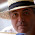## 16 August 2014

### Quiz 12: Find number of elements common to all chemicals

Problem:
An element may be represented by any lower case alphabet from a-z.
A chemical composition may contains one or more than one elements like a, aaz, abcddyy can be chemical composition

Input: A number N which represents number of chemicals followed by composition of chemicals

Output: Number of elements which are common to all given chemicals

Sample Input:
4
agmagmdddz
werfkjabbfmgaap
mgaaaqqqqqqagm
uaimpg

Sample Output:
3

constraints:
1<=N<=100
compositions can contain only lower case alphabets
1<=length of chemical composition<=100

Explanations:- 3 elements a,g and m are present in all chemical compositions, so output is 3

Solution:

chomp(\$n=<STDIN>);
if(\$n<1 or \$n>100)
{
exit;
}
@arr1=();
@arr3=();
\$count = 0;
@arr6 = qw(0 0 0 0 0 0 0 0 0 0 0 0 0 0 0 0 0 0 0 0 0 0 0 0 0 0);
for(\$i=0;\$i<\$n;\$i++)
{
chomp(\$comp=<STDIN>);
@arr1 = split("",\$comp);
if(@arr1 < 1 or @arr1 > 100)
{
exit;
}
foreach(@arr1)
{
\$asc=ord("\$_");
if(\$asc < 97 or \$asc > 122)
{
exit;
}
}
\$all = "abcdefghijklmnopqrstuvwxyz";
\$a = \$all.\$comp;
@arr2 = split("",\$a);
@arr2 = sort(@arr2);
\$a = join("",@arr2);
my @arr = split("",\$a);
my \$tmp = 0;
for(my \$i=0;\$i<@arr;\$i++)
{
if(\$arr[\$i] eq \$arr[\$i+1])
{
if(\$tmp < 1)
{
\$tmp++;
}
}
else{
push(@arr3,\$arr[\$i].\$tmp);
\$tmp = 0;
}
}
\$c = ":";
push(@arr3,\$c);
}
\$b = join("",@arr3);
@arr4 = split(/:/,\$b);
foreach(@arr4)
{
@arr9 = split(/\D/,\$_);
for (\$i=0;\$i<@arr6;\$i++){
\$arr6[\$i] = \$arr6[\$i] + \$arr9[\$i+1];
}
}
foreach(@arr6)
{
if(\$_ eq \$n)
{
\$count++;
}
}
print "\$count";

Tips:
• ord is used to get ascii values

1.Wow. Your solution is *really* over-complicated!

#!/usr/bin/perl

use strict;
use warnings;

chomp(my \$n = );

my @input;
for (1 .. \$n) {
push @input, ;
}
chomp @input;

# Clean up input so each string only contains
# each letter once
@input = map {
my %seen;
\$seen{\$_}++ for split //;
join '', keys %seen;
} @input;

# Count the occurences of letters across all
# strings
my %seen;
foreach my \$ch (@input) {
\$seen{\$_}++ for split //, \$ch;
}

# If a letter appears in all input strings, then
# \$seen{\$letter} will equal \$n
my \$output;
foreach (keys %seen) {
\$output++ if \$seen{\$_} == \$n;
}

print "\$output\n";

2.Hi Dave

your program is giving error at line 10.
Useless use of push with no values at line 10.
syntax error at line 6, near "= >"

3.Your blog software has stripped out angle brackets in a couple of places. If you're collecting code samples, then I suggest that you use a blog system that understands code and doesn't remove sections without warning :-/